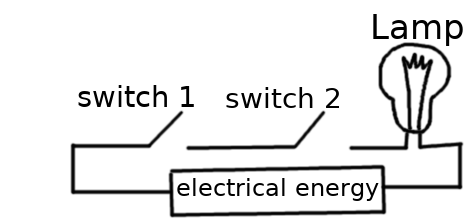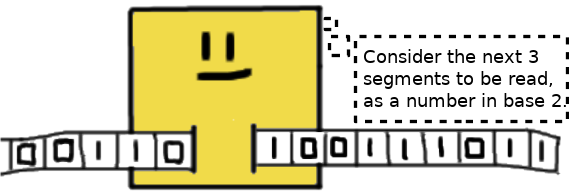# Creatures of X^n heads (part 1/5)

We humans are used to dealing with numbers using decimal notation, that is, ten symbols (0,1,2,3,4,5,6,7,8,9) that combined represent numerical quantities. In this system, the position of the symbol is important. For example, 23 and 32 use the same two symbols, but represent different things. We learned to perform calculations with numbers in the decimal number system. For example, to add the numbers 23 and 230 we need to adjust the position of the two numbers from right to left.

23
+230
253

No matter how many terms there are in this operation, we will have to adjust all numbers from right to left. For each position of the digit we know that we will work with ten different specific symbols. The ten symbols represent a standardization, a human convention. For humans, this system of operations could be called an creature with 10n heads, where 10 is the base, that is, the number of distinct symbols to compose any number and n is the exponent that represents how many different combinations (10n) we will obtain with the ten symbols.

However, the computer does not understand numbers as we do humans. In this way, the computer does not use the decimal numbering system internally. It uses a simpler system, with fewer symbols. Basically two symbols, 0 and 1. Again, 0 and 1 are accepted conventions. It could be any two symbols, but it looks like 0 and 1 is something easier to understand. A numbering system with only two symbols (21) is called a binary numbering system. A number in this system would be something like:

1011011000111

I believe that for most of us, the binary base was a horror at first sight. But here is a secret, even though the decimal system seems much more intuitive and easier to use, this is perhaps a matter of custom because we were born in a world where this base reigns in most human communications. However, in the same way that in the decimal system we can perform mathematical operations, with binary numbers we can also do them, and this occurs in an extremely simple way, as it involves only rudimentary actions to operate 0’s and 1’s. Below, we exemplify some operations (addition, subtraction, multiplication and division) on this basis.

 101+111000 1011-100111 1101x11111011101+1101++1011011 101 |1110 1101

The branch of mathematics that deals with this numbering system with two symbols is known as Boolean Algebra and was created by 19th century mathematician, philosopher and logician, George Boole. The computer uses Boolean Algebra to perform its operations. Thus, the computer is a creature with 2n heads.

We can exemplify a 21 head computer as a light bulb connected to a switch. The switch (as the name implies) interrupts the electrical energy to reach the lamp. Thus, when we close the circuit (that is, we turn on the switch), the energy passes from that point and reaches the lamp, causing it to light. Similarly, by increasing the circuits connected with this lamp, we generate more options to be satisfied. Assuming the case of two switches connected in series, for the lamp to light, both the first and the second must have their electrical circuits closed, allowing energy to pass through them and reach the lamp.Electrical circuit connected in series.

Basically, all computers are gigantic circuits with complex switches that “turn lights on or off”. The ideology behind the modern computer is attributed to Alan M. Turing. In this case, Turing proposed a device that would read an infinite magnetic tape with its segments occupied (true value or 1) or free (false value or 0) and would decide from these actions: forward or rewind the tape, erase or mark segments of this tape with 0’s or 1’s. Although simple, this device became known as the Universal Turing Machine and there is a maxim in computer science, stating that such a conceptual device is capable of simulating the functioning of any computer.Representation of a Universal Turing Machine.